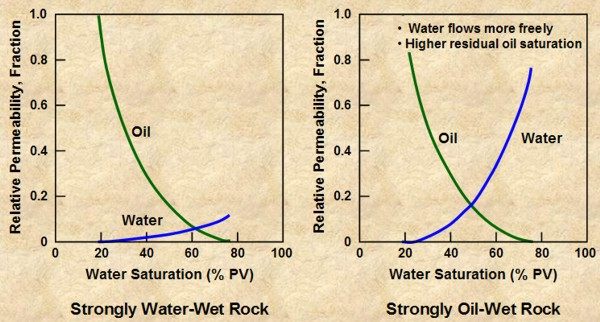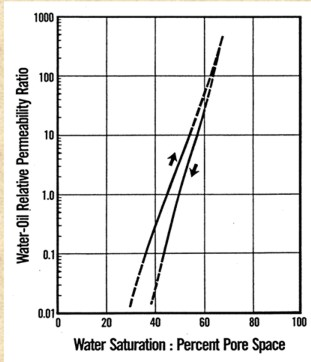RELATIVE PERMEABILITY
Relative permeability is the ratio of the effective permeability of a fluid at a given saturation to some base permeability. Base permeability is typically defined as absolute permeability (Ka), air permeability (Kair), or effective permeability to non-wetting phase at irreducible wetting phase saturation, for example Ko @ Sw = SWir. Because the definition of base permeability varies, the definition used must always be confirmed before applying relative permeability data noted along with tables and figures presenting relative permeability data.Relative permeability curves in water wet and oil wet reservoirsMEASURING RELATIVE PERMEABILITY
Stage 1:  Preparation for drainage - core saturated with wetting phase.

Stage 2: Irreducible wetting phase (drainage) -
inject non-wetting phase until steady state, measure saturation, no wetting phase will be produced after steady state.

Stages 3-6:  Inject wetting phaseStage 3: (A) Wetting phase has not yet reached outflow face, only nonwetting phase produced at outflow face.

•   Stage 4: (B) Wetting phase just reaches outflow face, called breakthrough, wetting phase will be produced at outflow face.

Stage 5: (C) As injection of wetting phase continues, production of nonwetting phase decreases (unsteady state). Important to take many data points during this decrease (»cummulative nonwetting phase produced »and production rate for both phases).

Stage 6: (D) Eventually, no more nonwetting phase is produced, allowing residual nonwetting phase saturation to be determined.Assumptions:
immiscible displacement
incompressible fluids
linear, 1-D flow
capillary pressure neglectedAnalysis Procedure:
Determine average saturation and

cumulative nonwetting phase production,
Determine relative permeability ratio from fractional flow (production rate measured at outflow face):
4: Qtotal = Qwet + Qnonwet
5: Fwet = Qwet / Qtotal

Relative permeability ratio Fwet is related to relative permeability by the equation:
6: Fwet = 1 / (1 + (Kr_nonwet / Kr_wet)
* (MUwet / MUnonwet))

It is obvious from the above that one of Kr_nonwet or Kr_wet must be determined independently in order to find the other, using Fwet. This is done with correlation equations based on capillary pressure curves.

The process starts with calculation of effective water saturation (not to be confused with any form of water saturation from log analysis).

7: So* = So / (1 - Swi)
8: Sw* = (Sw - Swi) / (1 - Swi)
9: Sg* = Sg / (1 - Swi)

Various authors have proposed a variety of relative permeability relationships based on the rock type. Eor example Pirson propsed:
10: Kr_wetting = (Sw*)^0.5 * (Sw)^3
11: Kr_nonwettimg = (1 - ((Sw - Swi) / (1 - Swi - Snw)))^2  for imbibition
12: Kr_nonwetting = (1 - Sw*) * (1 - (Sw*)^0.25 * (Sw)^0.5)^0.5 for drainage

Pretty messy but so is the math for the lab method.RELATIVE PERMEABILITY EXAMPLERELATIVE PERMEABILITY from MICRO CT SCANS
Relative permeability is a dimensionless measure of the permeability of a fluid phase as it flows through porous rock in the presence of another fluid phase.  Relative permeability is calculated as follows:If a single fluid is present in a rock, its relative permeability is 1.0. Relative permeability allows comparison of the different abilities of fluids to flow in the presence of each other, since the presence of more than one fluid generally inhibits flow.

Key parameters that affect relative permeability include:
• The pore-space geometry (the distribution of large and small conduits and their sizes)
• Viscosity of the fluids
• Wettability of the mineral surface, and
• The surface tension between the fluid phases and between each fluid phase and the minerals.
These parameters define the wetting (or contact) angles, which are formed at an interface between fluid and mineral. A wetting angle is larger than 90 degrees if the fluid is wetting and smaller than 90 degrees if the fluid is non-wetting.

The slow multiphase viscous flow needed for relative permeability estimates is simulated using the lattice Boltzmann method (LBM). LBM mathematically mimics the equations of multiphase viscous flow by treating the fluid as a set of particles with certain interaction rules between the particles belonging to the same fluid, different fluids, and the fluids and pore walls. LBM directly simulates static and dynamic configurations of the contacts between the fluid phases and the pore walls by taking into account surface tension and contact angles. It allows for the estimation of irreducible water and hydrocarbon saturations Source: www.ingrainrocks.com.Page Views ---- Since 01 Jan 2015